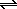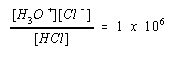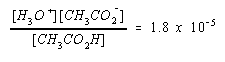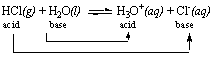Acid-Base Pairs, Strength of Acids and Bases, and pH

Acid-Base Pairs, Strength of Acids and Bases, and pH

Conjugate Acid-Base Pairs

Acids and bases exist as conjugate acid-base pairs. The term conjugate comes from the Latin stems meaning "joined together" and refers to things that are joined, particularly in pairs, such as Brnsted acids and bases.

Every time a Brnsted acid acts as an H+-ion donor, it forms a conjugate base. Imagine a generic acid, HA. When this acid donates an H+ ion to water, one product of the reaction is the A- ion, which is a hydrogen-ion acceptor, or Brnsted base.

 HA + H2OH3O+ + A- acid base

Conversely, every time a base gains an H+ ion, the product is a Brnsted acid, HA.

 A- + H2OHA + OH- base acid

Acids and bases in the Brnsted model therefore exist as conjugate pairs whose formulas are related by the gain or loss of a hydrogen ion.

Our use of the symbols HA and A- for a conjugate acid-base pair does not mean that all acids are neutral molecules or that all bases are negative ions. It signifies only that the acid contains an H+ ion that isn't present in the conjugate base. Brnsted acids or bases can be neutral molecules, positive ions, or negative ions. Various Brnsted acids and their conjugate bases are given in the table below.

Typical Brnsted Acids and Their Conjugate Bases

 Acid Base H3O+ H2O H2O OH- OH- O2- HCl Cl- H2SO4 HSO4- HSO4- SO42- NH4+ NH3 NH3 NH2-

A compound can be both a Brnsted acid and a Brnsted base. H2O, OH-, HSO4-, and NH3, for example, can be found in both columns in the table above. Water is the perfect example of this behavior because it simultaneously acts as an acid and a base when it forms the H3O+ and OH- ions.Strong and Weak Acids and Bases

Many hardware stores sell "muriatic acid"a 6 M solution of hydrochloric acid HCl(aq)to clean bricks and concrete. Grocery stores sell vinegar, which is a 1 M solution of acetic acid: CH3CO2H. Although both substances are acids, you wouldn't use muriatic acid in salad dressing, and vinegar is ineffective in cleaning bricks or concrete.

The difference between the two is that muriatic acid is a strong acid and vinegar is a weak acid. Muriatic acid is strong because it is very good at transferring an H+ ion to a water molecule. In a 6 M solution of hydrochloric acid, 99.996% of the HCl molecules react with water to form H3O+ and Cl- ions.

HCl(aq) + H2O(l)H3O+(aq) + Cl-(aq)

Vinegar is a weak acid because it is not very good at transferring H+ ions to water. In a 1 M solution, less than 0.4% of the CH3CO2H molecules react with water to form H3O+ and CH3CO2- ions.

CH3CO2H(aq) + H2O(l)H3O+(aq) + CH3CO2-(aq)

More than 99.6% of the acetic acid molecules remain intact.The relative strengths of acids is often described in terms of an acid-dissociation equilibrium constant, Ka. To understand the nature of this equilibrium constant, let's assume that the reaction between an acid and water can be represented by the following generic equation.

 HA(aq) + H2O(l)H3O+(aq) + A-(aq)

In other words,some of the HA molecules react to form H3O+ and A- ions, as shown in the figure below.By convention, the concentrations of these ions in units of moles per liter are represented by the symbols [H3O+] and [A-]. The concentration of the HA molecules that remain in solution is represented by the symbol [HA].

The value of Ka for acid is calculated from the following equation.When a strong acid dissolves in water, the acid reacts extensively with water to form H3O+ and A- ions. (Only a small residual concentration of the HA molecules remains in solution.) The product of the concentrations of the H3O+ and A- ions is therefore much larger than the concentration of the HA molecules, so Ka for a strong acid is greater than 1.

Example: Hydrochloric acid has a Ka of roughly 1 x 106.Weak acids, on the other hand, react only slightly with water. The product of the concentrations of the H3O+ and A- ions is therefore smaller than the concentration of the residual HA molecules. As a result, Ka for a weak acid is less than 1.

Example: Acetic acid has a Ka of only 1.8 x 10-5.Ka can therefore be used to distinguish between strong acids and weak acids.

 Strong acids: Ka > 1 Weak acids: Ka < 1The Relative Strengths of Conjugate Acid-base Pairs

• Strong acids have a weak conjugate base.

Example: HCl is a strong acid. If HCl is a strong acid, it must be a good proton donor. HCl can only be a good proton donor, however, if the Cl- ion is a poor proton acceptor. Thus, the Cl- ion must be a weak base.

 HCl(g) + H2O(l)H3O+(aq) + Cl-(aq) Strong acid Weak base
• Strong bases have a weak conjugate acid.

Example: Let's consider the relationship between the strength of the ammonium (NH4+) and its conjugate base, ammonia (NH3). The NH4+ ion is a weak acid because ammonia is a reasonably good base.

 NH4+(aq) + H2O(l)H3O+(aq) + NH3(aq) Weak acid Good base

Practice Problem 3:

Use the acid-dissociation equilibrium constants for the conjugate acids of these bases to predict whether the CH3CO2- ion or the OH - ion is the stronger base.

 CH3CO2H: Ka = 1.8 x 10-5 H2O: Ka = 1.8 x 10-16Comparing Relative Strengths of Pairs of Acids and Bases

The value of Ka for an acid can be used to decide whether it is a strong acid or a weak acid, in an absolute sense. It can also be used l to compare the relative strengths of a pair of acids.

Example: Consider HCl and the H3O+ ion.

 HCl Ka = 1 x 106 H3O+ Ka = 55

These Ka values suggest that both are strong acids, but HCl is a stronger acid than the H3O+ ion.

A high proportion of the HCl molecules in an aqueous solution reacts with water to form H3O+ and Cl- ions. The Brnsted theory suggests that every acid-base reaction converts an acid into its conjugate base and a base into its conjugate acid.There are two acids and two bases in this reaction. The stronger acid, however, is on the left side of the equation.

 HCl(g) + H2O(l)H3O+(aq) + Cl-(aq) stronger acid weaker acid

The general rules suggest that the stronger of a pair of acids must form the weaker of a pair of conjugate bases. The fact that HCl is a stronger acid than the H3O+ ion implies that the Cl- ion is a weaker base than water.

 Acid strength: HCl > H3O+ Base strength: Cl- < H2O

Thus, the equation for the reaction between HCl and water can be written as follows.

 HCl(g) + H2O(l)H3O+(aq) + Cl-(aq) stronger acid stronger base weaker acid weaker base

It isn't surprising that 99.996% of the HCl molecules in a 6 M solution react with water to give H3O+ ions and Cl- ions. The stronger of a pair of acids should react with the stronger of a pair of bases to form a weaker acid and a weaker base.

Let's look at the relative strengths of acetic acid and the H3O+ ion.

 CH3CO2H Ka = 1.8 x 10-5 H3O+ Ka = 55

The values of Ka for these acids suggest that acetic acid is a much weaker acid than the H3O+ ion, which explains why acetic acid is a weak acid in water. Once again, the reaction between the acid and water must convert the acid into its conjugate base and the base into its conjugate acid.But this time, the stronger acid and the stronger base are on the right side of the equation.

 CH3CO2H(aq) + H2O(l)H3O+(aq) + CH3CO2-(aq) weaker acid weaker base stronger acid stronger base

As a result, only a few of the CH3CO2H molecules actually donate an H+ ion to a water molecule to form the H3O+ and CH3CO2- ions.

 Practice Problem 4:NaNH2 reacts with water to give an aqueous solution of NaOH and NH3. NaNH2(s) + H2O(l)Na+(aq) + OH-(aq) + NH3(aq) Determine which is the stronger base, the NH2- or OH- ions. Click here to check your answer to Practice Problem 4

The magnitude of Ka can also be used to explain why some compounds that qualify as Brnsted acids or bases don't act like acids or bases when they dissolve in water. When the value of Ka for an acid is relatively large, the acid reacts with water until essentially all of the acid molecules have been consumed. Sulfuric acid (Ka = 1 x 103), for example, reacts with water until 99.9% of the H2SO4 molecules in a 1 M solution have lost a proton to form HSO4- ions.

H2SO4(aq) + H2O(l)H3O+(aq) + HSO4-(aq)

As Ka becomes smaller, the extent to which the acid reacts with water decreases.

As long as Ka for the acid is significantly larger than the value of Ka for water, the acid will ionize to some extent. Acetic acid, for example, reacts to some extent with water to form H3O+ and CH3CO2-, or acetate, ions.

CH3CO2H(aq) + H2O(l)H3O+(aq) + CH3CO2-(aq)

As the Ka value for the acid approaches the Ka for water, the compound becomes more like water in its acidity. Although it is still a Brnsted acid, it is so weak that we may be unable to detect this acidity in aqueous solution.

Some potential Brnsted acids are so weak that their Ka values are smaller than water's. Ammonia, for example, has a Ka of only 1 x 10-33. Although NH3 can be a Brnsted acid, because it has the potential to act as a hydrogen-ion donor, there is no evidence of this acidity when it dissolves in water.The Leveling Effect of Water

All strong acids and bases seem to have the same strength when dissolved in water, regardless of the value of Ka. This phenomenon is known as the leveling effect of waterthe tendency of water to limit the strength of strong acids and bases. We can explain this by noting that strong acids react extensively with water to form the H3O+ ion. More than 99% of the HCl molecules in hydrochloric acid react with water to form H3O+ and Cl- ions, for example,

HCl(g) + H2O(l)H3O+(aq) + Cl-(aq)

and more than 99% of the H2SO4 molecules in a 1 M solution react with water to form H3O+ ions and HSO4- ions.

H2SO4(aq) + H2O(l)H3O+(aq) + HSO4-(aq)

Thus, the strength of strong acids is limited by the strength of the acid (H3O+) formed when water molecules pick up an H+ ion.

A similar phenomenon occurs in solutions of strong bases. Strong bases react quantitatively with water to form the OH- ion. Once this happens, the solution cannot become any more basic. The strength of strong bases is limited by the strength of the base (OH-) formed when water molecules lose an H+ ion.The Brnsted definition of acids and bases offers many advantages over the Arrhenius and operational definitions.

• It expands the list of potential acids to include positive and negative ions, as well as neutral molecules.
• It expands the list of bases to include any molecule or ion with at least one pair of nonbonding valence electrons.
• It explains the role of water in acid-base reactions: Water accepts H+ ions from acids to form the H3O+ ion.
• It can be expanded to include solvents other than water and reactions that occur in the gas or solid phases.
• It links acids and bases into conjugate acid-base pairs.
• It can explain the relationship between the strengths of an acid and its conjugate base.
• It can explain differences in the relative strengths of a pair of acids or a pair of bases.
• It can explain the leveling effect of waterthe fact that strong acids and bases all have the same strength when dissolved in water.

Because of these advantages, whenever chemists use the words acid or base without any further description, they are referring to a Brnsted acid or a Brnsted base.Pure water is both a weak acid and a weak base. By itself, water forms only a very small number of the H3O+ and OH- ions that characterize aqueous solutions of stronger acids and bases.

 H2O(l) + H2O(l)H3O+(aq) + OH-(aq) base acid acid base

The concentrations of the H3O+ and OH- ions in water can be determined by carefully measuring the ability of water to conduct an electric current. At 25oC, the concentrations of these ions in pure water is 1.0 x 10-7 moles per liter.

[H3O+] = [OH-] = 1.0 x 10-7 M (at 25C)

When we add a strong acid to water, the concentration of the H3O+ ion increases.

HCl(aq) + H2O(l)H3O+(aq) + Cl-(aq)

At the same time, the OH- ion concentration decreases because the H3O+ ions produced in this reaction neutralize some of the OH- ions in water.

H3O+(aq) + OH-(aq)2 H2O(l)

The product of the concentrations of the H3O+ and OH- ions is constant, no matter how much acid or base is added to water. In pure water at 25oC, the product of the concentration of these ions is 1.0 x 10-14.

[H3O+][OH-] = 1.0 x 10-14

The range of concentrations of the H3O+ and OH- ions in aqueous solution is so large that it is difficult to work with. In 1909 the Danish biochemist S. P. L. Sorenson suggested reporting the concentration of the H3O+ ion on a logarithmic scale, which he named the pH scale. Because the H3O+ ion concentration in water is almost always smaller than 1, the log of these concentrations is a negative number. To avoid having to constantly work with negative numbers, Sorenson defined pH as the negative of the log of the H3O+ ion concentration.

pH = -log [H3O+]

 Practice problem 5:Calculate the pH of Pepsi Cola if the concentration of the H3O+ ion in this solution is 0.00347 M. Click here to check your answer to Practice Problem 5 Click here to see a solution to Practice Problem 5

The concept of pH compresses the range of H3O+ ion concentrations into a scale that is much easier to handle. As the H3O+ ion concentration decreases from roughly 100 to 10-14, the pH of the solution increases from 0 to 14.

If the concentration of the H3O+ ion in pure water at 25oC is 1.0 x 10-7 M, the pH of pure water is 7.

pH = -log [H3O+] = -log (1.0 x 10-7) = 7

When the pH of a solution is less than 7, the solution is acidic. When the pH is more than 7, the solution is basic.

 Acidic: pH < 7 Basic: pH > 7pH of Common Acids and Bases

The pH of a solution depends on the strength of the acid or base in the solution. Measurements of the pH of dilute solutions are therefore good indicators of the relative strengths of acids and bases. Values of the pH of 0.10 M solutions of a number of common acids and bases are given in the table below.

pH of 0.10 M Solutions of Common Acids and Bases

 Compound pH HCl (hydrochloric acid) 1.1 H2SO4 (sulfuric acid) 1.2 NaHSO4 (sodium hydrogen sulfate) 1.4 H2SO3 (sulfurous acid) 1.5 H3PO4 (phosphoric acid) 1.5 HF (hydrofluoric acid) 2.1 CH3CO2H (acetic acid) 2.9 H2CO3 (carbonic acid) 3.8 (saturated solution) H2S (hydrogen sulfide) 4.1 NaH2PO4 (sodium dihydrogen phosphate) 4.4 NH4Cl (ammonium chloride) 4.6 HCN (hydrocyanic acid) 5.1 Na2SO4 (sodium sulfate) 6.1 NaCl (sodium chloride) 6.4 NaCH3CO2 (sodium acetate) 8.4 NaHCO3 (sodium bicarbonate) 8.4 Na2HPO4 (sodium hydrogen phosphate) 9.3 Na2SO3 (sodium sulfite) 9.8 NaCN (sodium cyanide) 11.0 NH3 (aqueous ammonia) 11.1 Na2CO3 (sodium carbonate) 11.6 Na3PO4 (sodium phosphate) 12.0 NaOH (sodium hydroxide, lye) 13.0